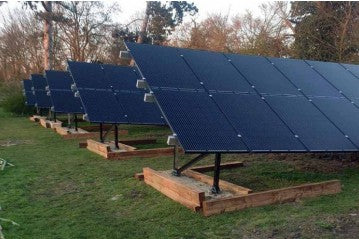# How Long Will a 12v Battery Last with Inverter?

Solar power systems are becoming increasingly popular as a sustainable and efficient energy solution. These systems typically consist of solar panels, solar charger controllers, solar batteries, and inverters. Solar batteries store the energy generated by the solar panels for later use, and the inverter converts this stored energy into usable AC power. If you're using a 12V battery with an inverter in your solar setup, it's important to understand how long the battery will last. Several factors come into play when determining the run time of a 12V battery with an inverter. Let's explore these factors and learn how to calculate the battery's running time.

## 1. Three Factors That Affect the Run Time of a 12V Battery with an Inverter

When estimating the battery's run time, three main factors need to be considered:

(1) How Many Watts Are in a 12-Volt Battery?

Watts are a unit of power and represent the rate at which energy is consumed or produced. To determine how long a 12V battery will last with an inverter, it's essential to know the wattage capacity of the battery. This capacity varies depending on the battery's size and specifications. A higher wattage capacity indicates that the battery can provide power for a more extended period.

(2) Depth of Discharge of the Battery

The depth of discharge refers to the amount of energy taken from the battery compared to its total capacity. Different battery types have varying discharge depths. Lead-acid batteries, for example, should not be discharged below 50% to ensure their longevity. Lithium-ion batteries, on the other hand, can safely handle deeper discharges, often up to 80% or more. Understanding the discharge depth of your specific battery is crucial when calculating its running time.

(3) Power Consumption of the Inverter from the Battery

To calculate how long a 12V battery will last with an inverter, you need to determine the total power consumption of the inverter and the loads connected to it in watts. The overall power consumption of an inverter can be divided into two parts: the no-load consumption of the inverter and the power consumption of the connected load.

• No-load consumption of the Inverter: This refers to the power consumed by the inverter itself when there is no load connected. It represents the energy required to run the inverter's internal circuitry.

• Power Consumption of the Load: This accounts for the power consumed by the devices or appliances connected to the inverter. Each device has its power requirements, which should be summed up to calculate the total load power.

Overall Power Consumption = Power Required by Load / Efficiency of Inverter + No-load Power Consumption of Inverter

By understanding these three factors and accurately calculating the power consumption, you can estimate the run time of a 12V battery with an inverter more effectively.

## 2. Two Steps to Calculate How Long a 12-Volt Battery Will Last with an Inverter

To determine the battery's running time, follow these two steps:

(1) Calculate the Battery Power Capacity

Start by identifying the power capacity of your 12V battery. This information is typically provided by the battery manufacturer and is measured in watt-hours (Wh) or ampere-hours (Ah). If the capacity is given in ampere-hours, multiply it by the battery voltage (12V) to obtain the capacity in watt-hours.

For example, if your battery has a capacity of 100Ah:
Battery Power Capacity = 100Ah x 12V = 1200Wh

(2) Calculate the Battery Running Time

To calculate the running time, divide the battery's power capacity by the total power consumption of the inverter and connected load. This will give

you the estimated running time in hours.

Running Time = Battery Power Capacity / Overall Power Consumption

By following these steps, you can get an estimate of how long your 12V battery will last with an inverter based on your specific power requirements.

## 3. Conclusion

Knowing how long a 12V solar battery will last with an inverter is crucial for properly sizing your solar power system and ensuring uninterrupted power supply. Factors such as the battery's wattage capacity, depth of discharge, and power consumption of the inverter and connected load all play significant roles in determining the battery's running time. By accurately calculating the battery's power capacity and considering the power requirements, you can make informed decisions when designing and using your solar setup.

Remember that different battery chemistries, such as lead-acid or lithium-ion, may have different discharge characteristics and efficiencies. Consult the manufacturer's specifications and guidelines for your specific battery to ensure optimal performance and longevity. By carefully considering these factors and performing the necessary calculations, you can maximize the efficiency and reliability of your solar power system with a 12V battery and inverter setup.

### Ein Kommentar hinterlassen

Alle Kommentare auf dem Blog werden vor der Veröffentlichung überprüft